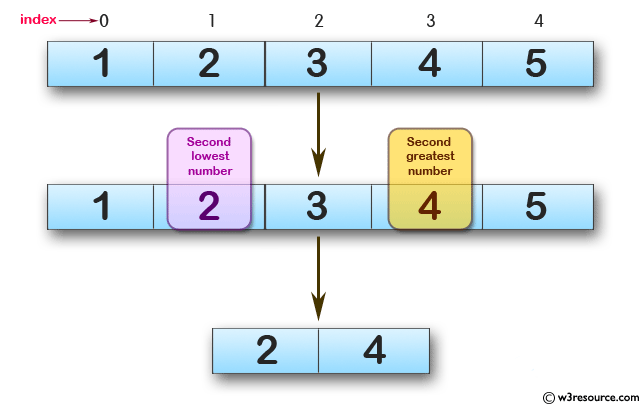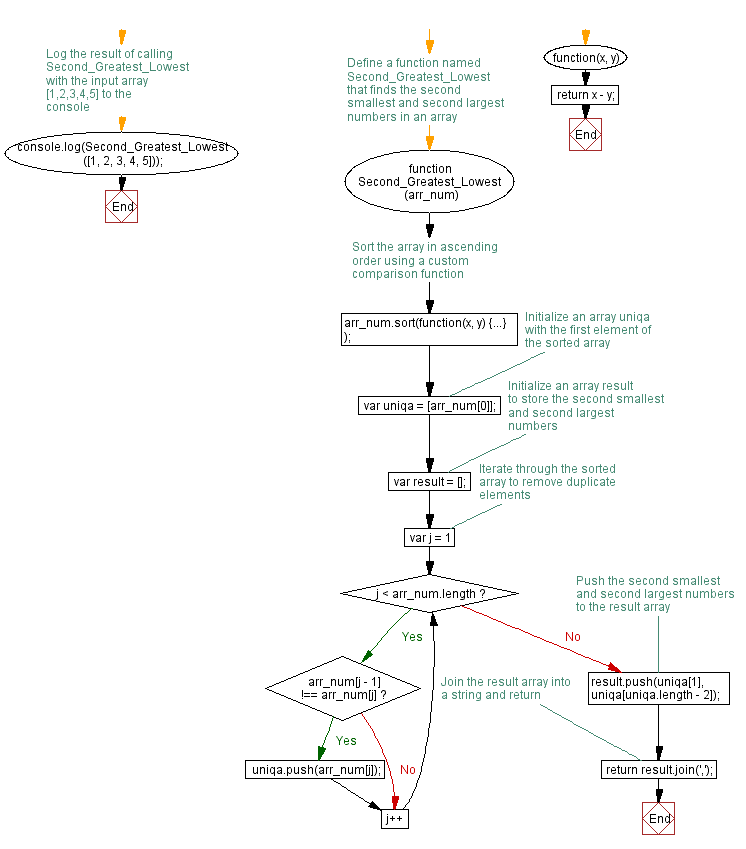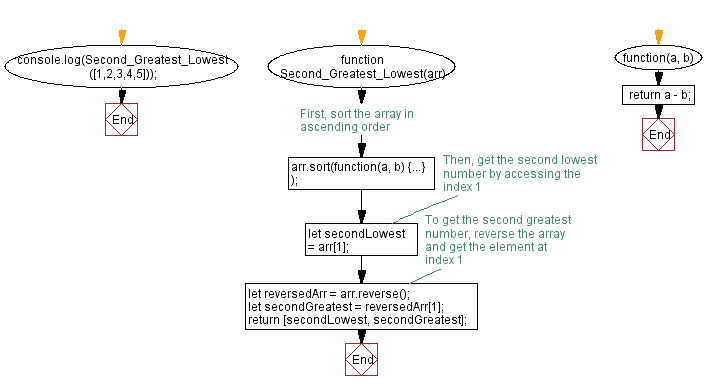# JavaScript: Find the second lowest and second greatest numbers from an array

## JavaScript Function: Exercise-11 with Solution

Write a JavaScript function that takes an array of numbers and finds the second lowest and second greatest numbers, respectively.

Pictorial Presentation:Sample Solution-1:

JavaScript Code:

``````function Second_Greatest_Lowest(arr_num)
{
arr_num.sort(function(x,y)
{
return x-y;
});
var uniqa = [arr_num];
var result = [];

for(var j=1; j < arr_num.length; j++)
{
if(arr_num[j-1] !== arr_num[j])
{
uniqa.push(arr_num[j]);
}
}
result.push(uniqa,uniqa[uniqa.length-2]);
return result.join(',');
}

console.log(Second_Greatest_Lowest([1,2,3,4,5]));
```
```

Sample Output:

``` 2,4
```

Flowchart:Live Demo:

See the Pen JavaScript -Second lowest and second greatest numbers from an array-function-ex- 11 by w3resource (@w3resource) on CodePen.

Improve this sample solution and post your code through Disqus

Sample Solution-2:

JavaScript Code:

``````function Second_Greatest_Lowest(arr) {
// First, sort the array in ascending order
arr.sort(function(a, b) {
return a - b;
});

// Then, get the second lowest number by accessing the index 1
let secondLowest = arr;

// To get the second greatest number, reverse the array and get the element at index 1
let reversedArr = arr.reverse();
let secondGreatest = reversedArr;

return [secondLowest, secondGreatest];
}
console.log(Second_Greatest_Lowest([1,2,3,4,5]));
```
```

Sample Output:

``` 2,4
```

Explanation:
The above function first sorts the input array in ascending order using the sort() method with a comparison function. Then, it retrieves the second lowest number in the array by accessing the element at index 1. The function reverses the sorted array to retrieve the element at index 1 of the reversed array, which is the second greatest number. Finally, the function returns an array containing the second lowest and second greatest numbers.

Flowchart:Live Demo:

See the Pen javascript-function-exercise-11-1 by w3resource (@w3resource) on CodePen.

Improve this sample solution and post your code through Disqus

What is the difficulty level of this exercise?

Test your Programming skills with w3resource's quiz.

﻿